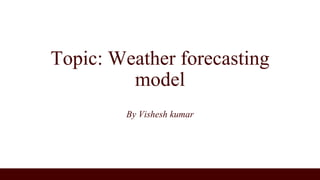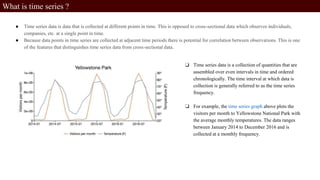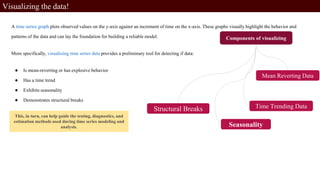Se está descargando tu SlideShare. ×

# Weather forecasting model.pptx

Anuncio
Anuncio
Anuncio
Anuncio
Anuncio
Anuncio
Anuncio
Anuncio
Anuncio
Anuncio
AnuncioCargando en…3
×

1 de 14 Anuncio

# Weather forecasting model.pptx

This is the weather forecasting model prepared considering the dataset of Newyork City for two weeks.

This is the weather forecasting model prepared considering the dataset of Newyork City for two weeks.

Anuncio
Anuncio

### Weather forecasting model.pptx

1. 1. Topic: Weather forecasting model By Vishesh kumar
2. 2. What is time series ? ● Time series data is data that is collected at different points in time. This is opposed to cross-sectional data which observes individuals, companies, etc. at a single point in time. ● Because data points in time series are collected at adjacent time periods there is potential for correlation between observations. This is one of the features that distinguishes time series data from cross-sectional data. ❏ Time series data is a collection of quantities that are assembled over even intervals in time and ordered chronologically. The time interval at which data is collection is generally referred to as the time series frequency. ❏ For example, the time series graph above plots the visitors per month to Yellowstone National Park with the average monthly temperatures. The data ranges between January 2014 to December 2016 and is collected at a monthly frequency.
3. 3. Visualizing the data! A time series graph plots observed values on the y-axis against an increment of time on the x-axis. These graphs visually highlight the behavior and patterns of the data and can lay the foundation for building a reliable model. More specifically, visualizing time series data provides a preliminary tool for detecting if data: ● Is mean-reverting or has explosive behavior ● Has a time trend ● Exhibits seasonality ● Demonstrates structural breaks This, in turn, can help guide the testing, diagnostics, and estimation methods used during time series modeling and analysis. Seasonality Structural Breaks Mean Reverting Data Time Trending Data
4. 4. Mean Reverting Data ❏ Mean reverting data returns, over time, to a time-invariant mean. It is important to know whether a model includes a non-zero mean because it is a prerequisite for determining appropriate testing and modeling methods. ❏ For example, unit root tests use different regressions, statistics, and distributions when a non-zero constant is included in the model. ❏ A time series graph provides a tool for visually inspecting if the data is mean-reverting, and if it is, what mean the data is centered around. While visual inspection should never replace statistical estimation, it can help you decide whether a non-zero mean should be included in the model. ❏ For example, the data in the figure above varies around a mean that lies above the zero line. This indicates that the models and tests for this data must incorporate a non-zero mean.
5. 5. Time Trending Data ❏ Time series data may also have a deterministic component that is proportionate to the time period. When this occurs, the time series data is said to have a time trend. ❏ Time trends in time series data also have implications for testing and modeling. The reliability of a time series model depends on properly identifying and accounting for time trends. ❏ A time series plot which looks like it centers around an increasing or decreasing line, like that in the plot above, suggests the presence of a time trend.
6. 6. Seasonality ❏ Seasonality is another characteristic of time series data that can be visually identified in time series plots. Seasonality occurs when time series data exhibits regular and predictable patterns at time intervals that are smaller than a year. ❏ An example of a time series with seasonality is retail sales, which often increase between September to December and will decrease between January and February.
7. 7. Structural Breaks ❏ Sometimes time series data shows a sudden change in behavior at a certain point in time. For example, many macroeconomic indicators changed sharply in 2008 after the start of the global financial crisis. These sudden changes are often referred to as structural breaks or non-linearities. ❏ These structural breaks can create instability in the parameters of a model. This, in turn, can diminish the validity and reliability of that model. ❏ Though statistical methods and tests should be used to test for structural breaks, time series plots can help for preliminary identification of structural breaks in data. ❏ Structural breaks in the mean of a time series will appear in graphs as sudden shifts in the level of the data at certain breakpoints. For example, in the time series plot above there is a clear jump in the mean of the data which around the start of 1980.
8. 8. Time Series and Stationarity ❏ A time series is stationary when all statistical characteristics of that series are unchanged by shifts in time. ❏ This is not to imply that stationarity is not an important concept in time series analysis. Many time series models are valid only under the assumption of weak stationarity ❏ Weak stationarity, henceforth stationarity, requires only that: ● A series has the same finite unconditional mean and finite unconditional variance at all time periods. ● That the series autocovariances are independent of time. ● Nonstationary time series are any data series that do not meet the conditions of a weakly stationary time series.
9. 9. Examples of Stationary Data
10. 10. Examples of Non-Stationary Data
11. 11. Time Series and Seasonality It is important to recognize the presence of seasonality in time series. Failing to recognize the regular and predictable patterns of seasonality in time series data can lead to incorrect models and interpretations. There are many tools that are useful for detecting seasonality in time series data: ● Background theory and knowledge of the data can provide insight into the presence and frequency of seasonality. ● Time series plots such as the seasonal subseries plot, the autocorrelation plot, or a spectral plot can help identify obvious seasonal trends in data. ● Statistical analysis and tests, such as the autocorrelation function, periodograms, or power spectrums can be used to identify the presence of seasonality. Dealing With Seasonality in Time Series Data Once seasonality is identified, the proper steps must be taken to deal with its presence. There are a few options for addressing seasonality in time series data: ● Choose a model that incorporates seasonality, like the Seasonal Autoregressive Integrated Moving Average (SARIMA) models. ● Remove the seasonality by seasonally detrending the data or smoothing the data using an appropriate filter. If the model is going to be used for forecasting, the seasonal component must be included in the forecast. ● Use a seasonally adjusted version of the data. For example, the Bureau of Labor Statistics provides U.S. labor and employment data and offers many series in both seasonally adjusted and not- seasonally adjusted formats.
12. 12. Time Series and Autocorrelation In time series data, autocorrelation is the correlation between observations of the same dataset at different points in time. The need for distinct time series models stems in part from the autocorrelation present in time series data.
13. 13. What Is an ARIMA Model? The autoregressive integrated moving average model (ARIMA) is a fundamental univariate time series model. The ARIMA model is made up of three key components: ● The autoregressive component is the relationship between the current dependent variable the dependent variable at lagged time periods. ● The integrated component refers to the use of transforming the data by subtracting past values of a variable from the current values of a variable in order to make the data stationary. ● The moving average component refers to the dependency between the dependent variable and past values of a stochastic term.
14. 14. The ARIMA data is described by the order of each of these components with the notation ARIMA(p, d, q) where: ● p is the number of autoregressive lags included in the model. ● d is the order of differencing used to make the data stationary. ● q is the number of moving average lags included in the model. What Is the Box-Jenkins Method for ARIMA Models? The Box-Jenkins method for estimating ARIMA models is made up of several steps: ● Transform data so it meets the assumption of stationarity. ● Identify initial proposals for p, d, and q. ● Estimate the model using the proposed p, d, and q. ● Evaluate the performance of the proposed p, d, and q. ● Repeat steps 2-4 as needed to improve model fit.